# hist3

Bivariate histogram plot

## Syntax

``hist3(X)``
``hist3(X,'Nbins',nbins)``
``hist3(X,'Ctrs',ctrs)``
``hist3(X,'Edges',edges)``
``hist3(___,Name,Value)``
``hist3(ax,___)``
``N = hist3(___)``
``[N,c] = hist3(___)``

## Description

example

````hist3(X)` creates a bivariate histogram plot of `X(:,1)` and `X(:,2)` using 10-by-10 equally spaced bins. The `hist3` function displays the bins as 3-D rectangular bars, and the height of each bar indicates the number of elements in the bin.```

example

````hist3(X,'Nbins',nbins)` specifies the number of bins in each dimension of the histogram. This syntax is equivalent to `hist3(X,nbins)`.```

example

````hist3(X,'Ctrs',ctrs)` specifies the centers of the bins in each dimension of the histogram. This syntax is equivalent to `hist3(X,ctrs)`.```
````hist3(X,'Edges',edges)` specifies the edges of the bins in each dimension.```

example

````hist3(___,Name,Value)` specifies graphical properties using one or more name-value pair arguments in addition to the input arguments in the previous syntaxes. For example, `'FaceAlpha',0.5` creates a semitransparent histogram. For a list of properties, see Surface Properties.```
````hist3(ax,___)` plots into the axes specified by `ax` instead of the current axes (`gca`). The option `ax` can precede any of the input argument combinations in the previous syntaxes.```

example

````N = hist3(___)` returns the number of elements in `X` that fall in each bin. This syntax does not create a histogram.```
````[N,c] = hist3(___)` also returns the bin centers. This syntax does not create a histogram.```

## Examples

collapse all

`load carbig`

Create a bivariate histogram with the default settings.

```X = [MPG,Weight]; hist3(X) xlabel('MPG') ylabel('Weight')```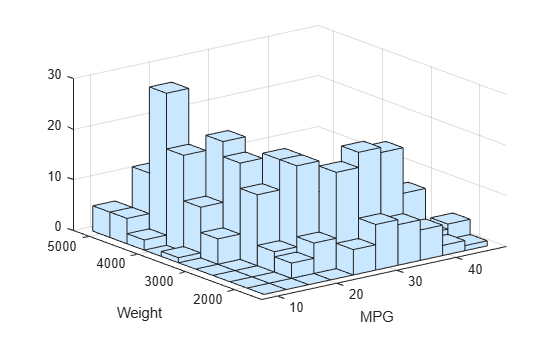Create a bivariate histogram on the bins specified by the bin centers, and count the number of elements in each bin.

`load carbig`

Create a bivariate histogram. Specify the centers of the histogram bins using a two-element cell array.

```X = [MPG,Weight]; hist3(X,'Ctrs',{0:10:50 2000:500:5000}) xlabel('MPG') ylabel('Weight')```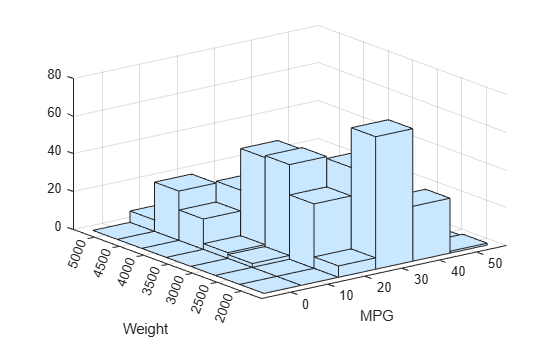Count the number of elements in each bin.

`N = hist3(X,'Ctrs',{0:10:50 2000:500:5000})`
```N = 6×7 0 0 0 0 0 0 0 0 0 2 3 16 26 6 6 34 50 49 27 10 0 70 49 11 3 0 0 0 29 4 2 0 0 0 0 1 0 0 0 0 0 0 ```

`load carbig`

Create a bivariate histogram. Specify graphical properties to color the histogram bars by height representing the frequency of the observations.

```X = [MPG,Weight]; hist3(X,'CDataMode','auto','FaceColor','interp') xlabel('MPG') ylabel('Weight')```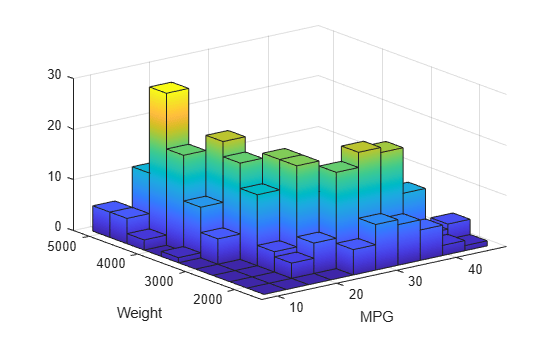`load carbig`

Create a bivariate tiled histogram. Specify graphical properties to color the top surface of the histogram bars by the frequency of the observations. Change the view to two-dimensional.

```X = [MPG,Weight]; hist3(X,'CdataMode','auto') xlabel('MPG') ylabel('Weight') colorbar view(2)```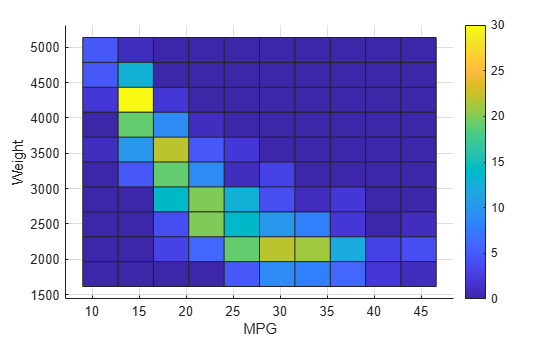Create a bivariate histogram and adjust its graphical properties by using the handle of the histogram surface object.

`load carbig`

Create a bivariate histogram with 7 bins in each dimension.

```X = [MPG,Weight]; hist3(X,'Nbins',[7 7]) xlabel('MPG') ylabel('Weight')```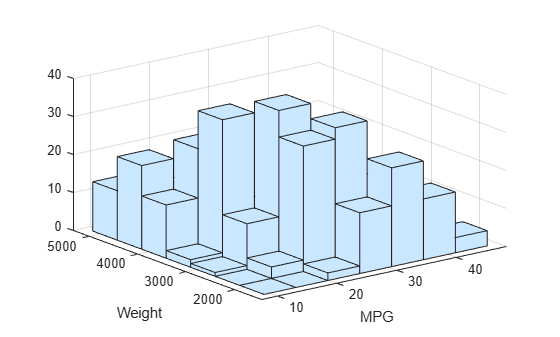The `hist3` function creates a bivariate histogram, which is a type of surface plot. Find the handle of the surface object and adjust the face transparency.

```s = findobj(gca,'Type','Surface'); s.FaceAlpha = 0.65;```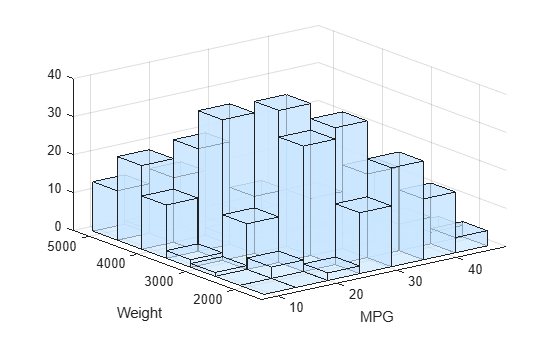Create a bivariate histogram and add the 2-D projected view of intensities to the histogram.

Load the `seamount` data set (a seamount is an underwater mountain). The data set consists of a set of longitude (`x`) and latitude (`y`) locations, and the corresponding `seamount` elevations (`z`) measured at those coordinates. This example uses `x` and `y` to draw a bivariate histogram.

`load seamount`

Draw a bivariate histogram.

```hist3([x,y]) xlabel('Longitude') ylabel('Latitude') hold on```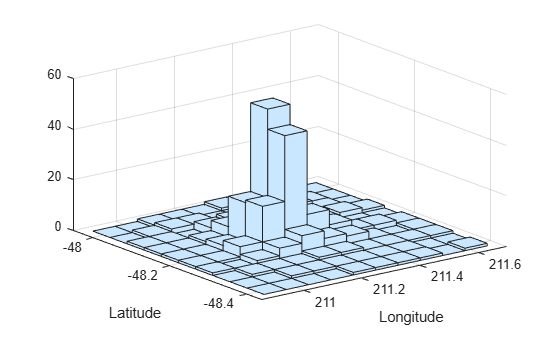Count the number of elements in each bin.

`N = hist3([x,y]);`

Generate a grid to draw the 2-D projected view of intensities by using `pcolor`.

```N_pcolor = N'; N_pcolor(size(N_pcolor,1)+1,size(N_pcolor,2)+1) = 0; xl = linspace(min(x),max(x),size(N_pcolor,2)); % Columns of N_pcolor yl = linspace(min(y),max(y),size(N_pcolor,1)); % Rows of N_pcolor```

Draw the intensity map by using `pcolor`. Set the z-level of the intensity map to view the histogram and the intensity map together.

```h = pcolor(xl,yl,N_pcolor); colormap('hot') % Change color scheme colorbar % Display colorbar h.ZData = -max(N_pcolor(:))*ones(size(N_pcolor)); ax = gca; ax.ZTick(ax.ZTick < 0) = []; title('Seamount Location Histogram and Intensity Map');```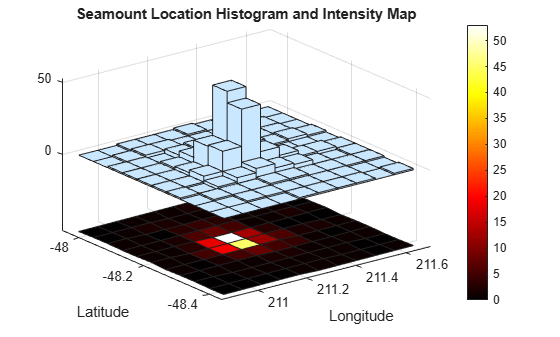## Input Arguments

collapse all

Data to distribute among the bins, specified as an m-by-2 numeric matrix, where m is the number of data points. Corresponding elements in `X(:,1)` and `X(:,2)` specify the x and y coordinates of 2-D data points.

`hist3` ignores all `NaN` values. Similarly, `hist3` ignores `Inf` and `–Inf` values unless you explicitly specify `Inf` or `–Inf` as a bin edge by using the `edges` input argument.

Data Types: `single` | `double`

Number of bins in each dimension, specified as a two-element vector of positive integers. `nbins(1)` specifies the number of bins in the first dimension, and `nbins(2)` specifies the number of bins in the second dimension.

Example: `[10 20]`

Data Types: `single` | `double`

Bin centers in each dimension, specified as a two-element cell array of numeric vectors with monotonically nondecreasing values. `ctrs{1}` and `ctrs{2}` are the positions of the bin centers in the first and second dimensions, respectively.

`hist3` assigns rows of `X` falling outside the range of the grid to the bins along the outer edges of the grid.

Example: `{0:10:100 0:50:500}`

Data Types: `cell`

Bin edges in each dimension, specified as a two-element cell array of numeric vectors with monotonically nondecreasing values. `edges{1}` and `edges{2}` are the positions of the bin edges in the first and second dimensions, respectively.

The value `X(k,:)` is in the `(i,j)`th bin if `edges{1}(i) ≤ X(k,1) < edges{1}(i+1)` and `edges{2}(j) ≤ X(k,2) < edges{2}(j+1)`.

The last bins in each dimension also include the last (outer) edge. For example, `X(k,:)` falls into the `(I,j)`th bin if `edges{1}(I–1) ≤ X(k,1) ≤ edges{1}(I)` and `edges{2}(j) ≤ X(k,2) < edges{2}(j+1)`, where `I` is the length of `edges{1}`. Also, `X(k,:)` falls into the `(i,J)`th bin if `edges{1}(i) ≤ X(k,1) < edges{1}(i+1)` and `edges{2}(J–1) ≤ X(k,2) ≤ edges{2}(J)`, where `J` is the length of `edges{2}`.

`hist3` does not count rows of `X` falling outside the range of the grid. Use `–Inf` and `Inf` in `edges` to include all non-`NaN` values.

Example: `{0:10:100 0:50:500}`

Data Types: `cell`

Target axes, specified as an axes object. If you do not specify an `Axes` object, then the `hist3` function uses the current axes (`gca`). For details, see Axes Properties.

### Name-Value Arguments

Specify optional comma-separated pairs of `Name,Value` arguments. `Name` is the argument name and `Value` is the corresponding value. `Name` must appear inside quotes. You can specify several name and value pair arguments in any order as `Name1,Value1,...,NameN,ValueN`.

Example: `hist3(X,'FaceColor','interp','CDataMode','auto')` colors the histogram bars according to the height of the bars.

The graphical properties listed here are only a subset. For a full list, see Surface Properties.

Selection mode for `CData` (vertex colors), specified as the comma-separated pair consisting of `'CDataMode'` and one of these values:

• `'manual'` — Use manually specified values in the `CData` property. The default color in `CData` is light steel blue corresponding to an RGB triple value of ```[0.75 0.85 0.95]```.

• `'auto'` — Use the `ZData` values to set the colors. `ZData` contains the z-coordinate data for the eight corners of each bar.

Example: `'CDataMode','auto'`

Edge line color, specified as the comma-separated pair consisting of `'EdgeColor'` and one of these values:

• `'none'` — Do not draw the edges.

• `'flat'` — Use a different color for each edge based on the values in the `CData` property.

• `'interp'` — Use interpolated coloring for each edge based on the values in the `CData` property.

• RGB triplet, hexadecimal color code, color name, or short name — Use the specified color for all the edges. These values do not use the color values in the `CData` property.

The default color of `[0 0 0]` corresponds to black edges.

RGB triplets and hexadecimal color codes are useful for specifying custom colors.

• An RGB triplet is a three-element row vector whose elements specify the intensities of the red, green, and blue components of the color. The intensities must be in the range `[0,1]`; for example,``` [0.4 0.6 0.7]```.

• A hexadecimal color code is a character vector or a string scalar that starts with a hash symbol (`#`) followed by three or six hexadecimal digits, which can range from `0` to `F`. The values are not case sensitive. Thus, the color codes `'#FF8800'`, `'#ff8800'`, `'#F80'`, and `'#f80'` are equivalent.

Alternatively, you can specify some common colors by name. This table lists the named color options, the equivalent RGB triplets, and hexadecimal color codes.

Color NameShort NameRGB TripletHexadecimal Color CodeAppearance
`'red'``'r'``[1 0 0]``'#FF0000'``'green'``'g'``[0 1 0]``'#00FF00'``'blue'``'b'``[0 0 1]``'#0000FF'``'cyan'` `'c'``[0 1 1]``'#00FFFF'``'magenta'``'m'``[1 0 1]``'#FF00FF'``'yellow'``'y'``[1 1 0]``'#FFFF00'``'black'``'k'``[0 0 0]``'#000000'``'white'``'w'``[1 1 1]``'#FFFFFF'`Here are the RGB triplets and hexadecimal color codes for the default colors MATLAB® uses in many types of plots.

`[0 0.4470 0.7410]``'#0072BD'``[0.8500 0.3250 0.0980]``'#D95319'``[0.9290 0.6940 0.1250]``'#EDB120'``[0.4940 0.1840 0.5560]``'#7E2F8E'``[0.4660 0.6740 0.1880]``'#77AC30'``[0.3010 0.7450 0.9330]``'#4DBEEE'``[0.6350 0.0780 0.1840]``'#A2142F'`Example: `'EdgeColor','blue'`

Face transparency, specified as the comma-separated pair consisting of `'FaceAlpha'` and one of these values:

• Scalar in the range `[0,1]` — Use uniform transparency across all the faces. A value of `1` is fully opaque and `0` is completely transparent. Values between `0` and `1` are semitransparent. This option does not use the transparency values in the `AlphaData` property.

• `'flat'` — Use a different transparency for each face based on the values in the `AlphaData` property. The transparency value at the first vertex determines the transparency for the entire face. This value applies only when you specify the `AlphaData` property and set the `FaceColor` property to `'flat'`.

• `'interp'` — Use interpolated transparency for each face based on the values in the `AlphaData` property. The transparency varies across each face by interpolating the values at the vertices. This value applies only when you specify the `AlphaData` property and set the `FaceColor` property to `'interp'`.

• `'texturemap'` — Transform the data in `AlphaData` so that it conforms to the surface.

Example: `'FaceAlpha',0.5`

Face color, specified as the comma-separated pair consisting of `'FaceColor'` and one of these values:

• `'flat'` — Use a different color for each face based on the values in the `CData` property.

• `'interp'` — Use interpolated coloring for each face based on the values in the `CData` property.

• `'none'` — Do not draw the faces.

• `'texturemap'` — Transform the color data in `CData` so that it conforms to the surface.

• RGB triplet, hexadecimal color code, color name, or short name — Use the specified color for all the faces. These values do not use the color values in the `CData` property.

RGB triplets and hexadecimal color codes are useful for specifying custom colors.

• An RGB triplet is a three-element row vector whose elements specify the intensities of the red, green, and blue components of the color. The intensities must be in the range `[0,1]`; for example,``` [0.4 0.6 0.7]```.

• A hexadecimal color code is a character vector or a string scalar that starts with a hash symbol (`#`) followed by three or six hexadecimal digits, which can range from `0` to `F`. The values are not case sensitive. Thus, the color codes `'#FF8800'`, `'#ff8800'`, `'#F80'`, and `'#f80'` are equivalent.

Alternatively, you can specify some common colors by name. This table lists the named color options, the equivalent RGB triplets, and hexadecimal color codes.

Color NameShort NameRGB TripletHexadecimal Color CodeAppearance
`'red'``'r'``[1 0 0]``'#FF0000'``'green'``'g'``[0 1 0]``'#00FF00'``'blue'``'b'``[0 0 1]``'#0000FF'``'cyan'` `'c'``[0 1 1]``'#00FFFF'``'magenta'``'m'``[1 0 1]``'#FF00FF'``'yellow'``'y'``[1 1 0]``'#FFFF00'``'black'``'k'``[0 0 0]``'#000000'``'white'``'w'``[1 1 1]``'#FFFFFF'`Here are the RGB triplets and hexadecimal color codes for the default colors MATLAB uses in many types of plots.

`[0 0.4470 0.7410]``'#0072BD'``[0.8500 0.3250 0.0980]``'#D95319'``[0.9290 0.6940 0.1250]``'#EDB120'``[0.4940 0.1840 0.5560]``'#7E2F8E'``[0.4660 0.6740 0.1880]``'#77AC30'``[0.3010 0.7450 0.9330]``'#4DBEEE'``[0.6350 0.0780 0.1840]``'#A2142F'`Example: `'FaceColor','interp'`

Line style, specified as the comma-separated pair consisting of `'LineStyle'` and one of the options in this table.

Line StyleDescriptionResulting Line
`'-'`Solid line`'--'`Dashed line`':'`Dotted line`'-.'`Dash-dotted line`'none'`No lineNo line

Example: `'LineStyle',':'`

Line width, specified as the comma-separated pair consisting of `'LineWidth'` and a positive value in points.

Example: `'LineWidth',0.75`

Data Types: `single` | `double`

## Output Arguments

collapse all

Number of elements in `X` that fall in each bin, returned as a numeric matrix.

Bin centers in each dimension, returned as a two-element cell array of numeric vectors. `c{1}` and `c{2}` are the positions of the bin centers in the first and second dimensions, respectively.

## Tips

The `hist3` function creates a bivariate histogram, which is a type of surface plot. You can specify surface properties using one or more name-value pair arguments. Also, you can change the appearance of the histogram by changing the surface property values after you create a histogram. Get the handle of the surface object by using `s = findobj(gca,'Type','Surface')`, and then use `s` to modify the surface properties. For an example, see Adjust Graphical Properties. For a list of properties, see Surface Properties.

## Alternative Functionality

The `histogram2` function enables you to create a bivariate histogram using a `Histogram2` object. You can use the name-value pair arguments of `histogram2` to use normalization (`'Normalization'`), adjust the width of the bins in each dimension (`'BinWidth'`), and display the histogram as a rectangular array of tiles instead of 3-D bars (`'DisplayStyle'`).